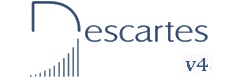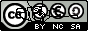First and Second Year of Secondary Education INDEX Introduction Objectives 1- Multiplication 2- Properties 3- Multiplications 4- Multiplications 5- Multiplications 6- Multiplications 7- Multiplications 8- Multiplications 9- Multiplications 10- Multiplications 11- Multiplications 1- Division 2- Divisions 3- Divisions 4- Divisions 5- Divisions 6- Divisions 7- Divisions 1- Powers 2- Square and cube 1- Combined Operations
Operations with integers II
INTRODUCCTION

In this unit, we revise the basic ideas of integers and we use them to practise the following operations: sum, multiplication, division and powers.
Before starting this unit, students should have studied integers in class in the traditional way. These activities are suitable as a complement to get a better understanding of the concepts and the operations.

The numbers that appear in each window are generated at random. This allows you to use the window indefinitely and practise a lot of different exercises. The numbers consist of only one or two figures so the calculations can easily be done mentally.

Magic squares with sums and multiplications are used in the exercises, however the main objective of this unit is to learn things about integers. It doesn't deal with learning things about magic squares.

OBJECTIVES
• To identify values which are integers.
• To understand the concept of multiplication of two integers.
• To take full advantage of the properties of multiplication.
• To understand how division is different from multiplication.
• To understand how powers is a repeated multiplication of the same factor.
• To understand and apply properly the hierarchy when combining different operations.
• To train in mental calculation.

 Eduardo Barbero CorralSpanish Ministry of Education. Year 2007